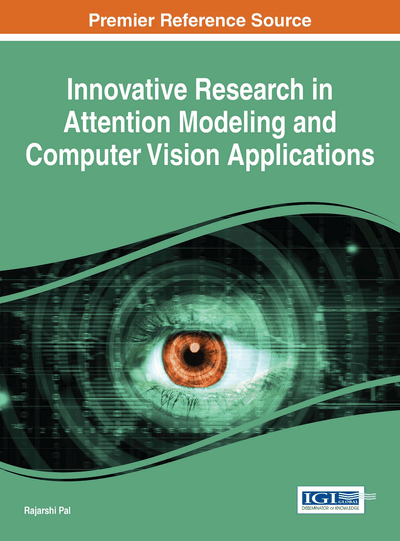# A Generic Design for Implementing Intersection between Triangles in Computer Vision and Spatial Reasoning

Chaman L. Sabharwal (Missouri University of Science and Technology, USA) and Jennifer L. Leopold (Missouri University of Science and Technology, USA)
DOI: 10.4018/978-1-4666-8723-3.ch008

## Abstract

The intersection between 3D objects plays a prominent role in spatial reasoning, and computer vision. Detection of intersection between objects can be based on the triangulated boundaries of the objects, leading to computing triangle-triangle intersection. Traditionally there are separate algorithms for cross and coplanar intersection. For qualitative reasoning, intersection detection is sufficient, actual intersection is not necessary; in contrast, the precise intersection is required for geometric modeling. Herein we present a complete design and implementation of a single integrated algorithm independent of the type of intersection. Additionally, this algorithm first detects, then intersects and classifies the intersections using barycentric coordinates. This work is directly applicable to: (1) VRCC-3D+, which uses intersection detection between 3D objects as well as their 2D projections essential for occlusion detection; and (2) CAD/CAM geometric modeling where curves of intersection between a pair of surfaces are required for numerical control machines. Experimental results are provided.
Chapter Preview
Top

## Introduction

Triangular mesh is used to represent the boundary of freeform 3D objects. The intersection between 3D objects plays a prominent role in spatial reasoning, geometric modeling, and computer vision. Detection of possible intersection between objects can be based on the objects’ boundaries (approximate triangulations of objects) in region connection calculi (RCC8), leading to computing triangle-triangle intersection. Traditionally there are specialized algorithms for cross intersection and coplanar intersection. The intersection detection is a byproduct of actual intersection computations. Most of the time intersection detection is done prior to the determination of the actual intersection so that the non-intersecting objects can be discarded. For example, in qualitative spatial reasoning, intersection detection is sufficient, actual intersection is not required; however in geometric applications, it is desirable to have actual intersections. For early intersection detection, we present a complete uniform integrated algorithm that is independent of cross and coplanar intersection. Herein we illustrate this with an algorithm, and with a Python implementation where the output is displayed with tables and figures.

The ability to detect the existence of possible intersection between pairs of objects is important in a variety of problem domains such as geographic information systems (Egenhofer & Golledge, 1998), real-time rendering (Tropp, Tal & Shimshoni, 2006), collision-detection, geology (Caumon, Collon, Le Carlier de Veslud, Viseur, & Sausse, 2009), surface modeling (Houghton, Emnett, Factor, & Sabharwal,1985), computer vision, networking and wireless computing. Typically, the boundary of each object is represented as a triangulated surface and a triangle-triangle intersection is the computational basis for determining intersection between objects. Since an object boundary may contain thousands of triangles, algorithms to speed up the intersection detection process are still being explored for various applications, sometimes with a focus on innovations in processor architecture (Elsheikh & Elsheikh, 2012).

For pairs of triangles, there are three types of intersections: zero dimensional (single point), one dimensional (line segment), and two dimensional (area) intersection. In the past, almost all attention has been devoted to determining the cross intersections, which resulted in an absence of analysis in two dimensional intersections. Coplanar triangle intersections are unique because an intersection may be any of the aforementioned three types. If the triangles cross intersect, only zero or one dimensional intersection is possible. If the planes are parallel and distinct, the triangles do not intersect. If the triangles are coplanar, then there is a possibility of intersection. Even when the cost of intersecting a triangle pair is constant, the cost of intersecting a pair of objects A and B is order O(TA* TB) where TA is the number of triangles in object A, and TB is the number of triangles in object B.

In qualitative spatial reasoning, spatial relations between regions are defined axiomatically using first order logic (Randell, Cui, & Cohn, 1992), or the 9-Intersection model (Egenhofer & Franzosa, 1991). Using the latter model, the spatial relations are defined using the intersections of the interior, boundary, and exterior of one region with those of a second region. It has been shown in (Sabharwal & Leopold, 2011) that it is sufficient to define the spatial relations by computing 4-Intersection predicates, (namely, Interior–Interior (IntInt), Boundary–Boundary (BndBnd), Interior–Boundary (IntBnd), and Boundary–Interior (BndInt)) instead of 9-Intersections. Since IntBnd and BndInt are the converse of each other, only three algorithms are necessary for these predicates. In order to implement these algorithms, we must first implement the triangle-triangle intersection determination. The triangle-triangle intersection is a lower level problem that must be solved in order to determine the 4-Intersection predicates which, in turn, determine the qualitative spatial relation between two objects.

## Complete Chapter List

Search this Book:
Reset